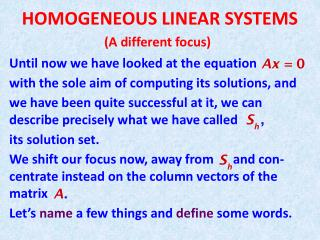Download PresentationHOMOGENEOUS LINEAR SYSTEMS# HOMOGENEOUS LINEAR SYSTEMS - PowerPoint PPT Presentation

Download Presentation##### HOMOGENEOUS LINEAR SYSTEMS

Download Policy: Content on the Website is provided to you AS IS for your information and personal use and may not be sold / licensed / shared on other websites without getting consent from its author. While downloading, if for some reason you are not able to download a presentation, the publisher may have deleted the file from their server.

- - - - - - - - - - - - - - - - - - - - - - - - - - - E N D - - - - - - - - - - - - - - - - - - - - - - - - - - -
##### Presentation Transcript

1. HOMOGENEOUS LINEAR SYSTEMS (A different focus) Until now we have looked at the equation with the sole aim of computing its solutions, and we have been quite successful at it, we can describe precisely what we have called its solution set. We shift our focus now, away from and con-centrate instead on the column vectors of the matrix Let’s name a few things and define some words.

2. We will call Obviously the fact that non-trivial solution says something about the vectors Not knowing what namely the following Definition. We say the set of vectors is linearly dependent if the equation non-trivial solution. Otherwise we say the set is linearly independent.

3. In order to follow the textbook, let’s turn the definition around and change the wording somewhat. Definition. We say the set of vectors is linearly independent if the vector equation has only the trivial solution We say that the set is linearly dependent if there are weights

4. A few remarks are in order. • Note how our old friends, the solutions have become • By abuse of notation, when the context is clear we will say that the vectors arelinearly dependent/independent rather than the set is linearly dependent/independent . • Let be the matrix with column vectors

5. Then the two statements are equivalent. (See statement on p. 67) This means that as far as verifying the linear dependence/independence of a set of vectors we are back to our old Let’s do an example. Are the three vectors

6. linearly independent? We row reduce the augmented matrix and conclude that they

7. Here are some interesting facts about linear dependence. • If the zero vector is part of the set of vectors then the set is linearly dependent. Proof: the set looks like Can you fill the blanks with at least one non-zero weight ? How about all zeroes, but In a formal proof I would write

8. Two non-zero vectors one is a multiple of the other. This is so trivial I am leaving out the proof. • If Proof. Think of the matrix , It has more columns than rows, therefore … the system

9. (Characterization of linear dependence) The set This is an important theorem (theorem 7, p. 58.) We will show the proof on the board. (and add it to the presentation later.)